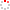#CodeStepByStep## contains

Language/Type: Java arrays traversals

Write a method named `contains` that accepts two arrays of integers a1 and a2 as parameters and that returns a boolean value indicating whether or not a2's sequence of elements appears in a1 (`true` for yes, `false` for no). The sequence of elements in a2 may appear anywhere in a1 but must appear consecutively and in the same order. For example, if variables called `a1` and `a2` store the following values:

```int[] a1 = {1, 6, 2, 1, 4, 1, 2, 1, 8};
int[] a2 = {1, 2, 1};
```

Then the call of `contains(a1, a2)` should return `true` because `a2`'s sequence of values `{1, 2, 1}` is contained in `a1` starting at index `5`. If `a2` had stored the values `{2, 1, 2}`, the call of `contains(a1, a2)` would return `false` because `a1` does not contain that sequence of values. Any two arrays with identical elements are considered to contain each other, so a call such as `contains(a1, a1)` should return `true`.

You may assume that both arrays passed to your method will have lengths of at least 1. You may not use any `String`s to help you solve this problem, nor methods that produce `String`s such as `Arrays.toString`.

Type your Java solution code here:

This is a method exercise. Write a Java method as described. Do not write a complete program or class; just the method(s) above.